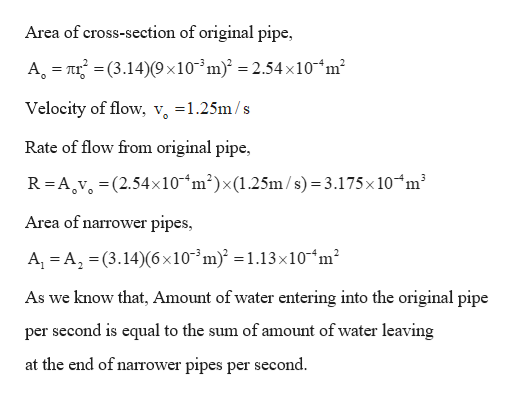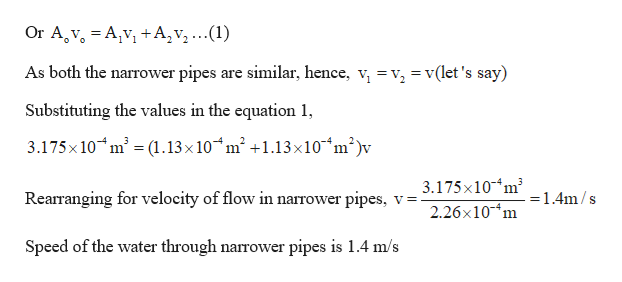# A man attaches a divider to an outdoor faucet so that water flows through a single pipe of radius 9.00 mm into two pipes, each with a radius of 6.00 mm. If water flows through the single pipe at 1.25 m/s, calculate the speed (in m/s) of the water in the narrower pipes.

Question
3 views

A man attaches a divider to an outdoor faucet so that water flows through a single pipe of radius 9.00 mm into two pipes, each with a radius of 6.00 mm. If water flows through the single pipe at 1.25 m/s, calculate the speed (in m/s) of the water in the narrower pipes.

check_circle

Step 1

Given:

Radius of the original pipe = 9 mm

Radius of the other two pipes = 6 mm

Velocity of flow in original pipe = 1.25 m/s

Step 2

Calculating the velocity of flow in other pipes:help_outlineImage TranscriptioncloseArea of cross-section of original pipe, A = nr =(3.14)(9 ×10-³m)² = 2.54x10-“m² Velocity of flow, v, =1.25m/s Rate of flow from original pipe, R= A,v, =(2.54×10“m²)×(1.25m /s) = 3.175x10ʻm³ Area of narrower pipes, A, = A, =(3.14)(6×10³m)² =1.13×10“m² As we know that, Amount of water entering into the original pipe per second is equal to the sum of amount of water leaving at the end of narrower pipes per second. fullscreen
Step 3

Calculating the speed of the flow ...help_outlineImage TranscriptioncloseOr A,v, = A,v, +A, v, ...(1) As both the narrower pipes are similar, hence, v, =v, =v(let 's say) Substituting the values in the equation 1, 3.175x 10 m = (1.13x10 m² +1.13x10*m²)v 3.175x10-m Rearranging for velocity of flow in narrower pipes, v= =1.4m/s 2.26x10-m Speed of the water through narrower pipes is 1.4 m/s fullscreen

### Want to see the full answer?

See Solution

#### Want to see this answer and more?

Solutions are written by subject experts who are available 24/7. Questions are typically answered within 1 hour.*

See Solution
*Response times may vary by subject and question.
Tagged in

### Fluid Mechanics